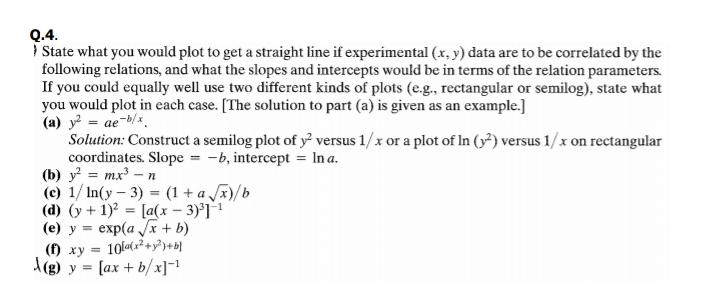# Q.4. I State what you would plot to get a straight line if experimental (x, y) data are to be correlated by the following relations, and what the slopes and intercepts would be in terms of the relation parameters. If you could equally well use two different kinds of plots (e.g., rectangular or semilog), state what you would plot in each case. [The solution to part (a) is given as an example.] (a) y = ae-b/. Solution: Construct a semilog plot of y versus 1/x or a plot of In (y) versus 1/x on rectangular coordinates. Slope = -b, intercept = In a. (b) y = mx-n (c) 1/ In(y- 3) = (1 + a x)/b (d) (y+1) = [a(x – 3)'] (e) y = exp(a x + b) () xy = 10+y A(g) y = [ax + b/x]=! %3D

Questionhelp_outlineImage TranscriptioncloseQ.4. I State what you would plot to get a straight line if experimental (x, y) data are to be correlated by the following relations, and what the slopes and intercepts would be in terms of the relation parameters. If you could equally well use two different kinds of plots (e.g., rectangular or semilog), state what you would plot in each case. [The solution to part (a) is given as an example.] (a) y = ae-b/. Solution: Construct a semilog plot of y versus 1/x or a plot of In (y) versus 1/x on rectangular coordinates. Slope = -b, intercept = In a. (b) y = mx-n (c) 1/ In(y- 3) = (1 + a x)/b (d) (y+1) = [a(x – 3)'] (e) y = exp(a x + b) () xy = 10+y A(g) y = [ax + b/x]=! %3D fullscreen

### Want to see this answer and more?

Experts are waiting 24/7 to provide step-by-step solutions in as fast as 30 minutes!*

*Response times may vary by subject and question complexity. Median response time is 34 minutes for paid subscribers and may be longer for promotional offers.
Tagged in
Math
Geometry

### Other